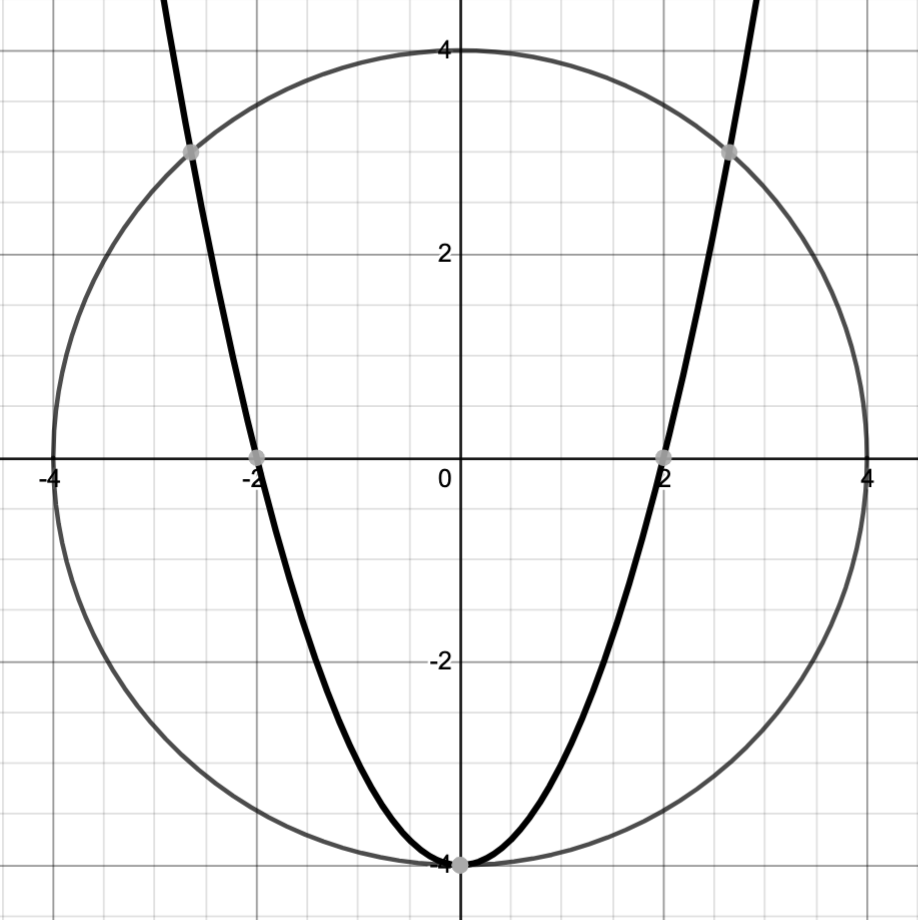### Home > CCA2 > Chapter 11 > Lesson 11.3.1 > Problem11-90

11-90.

Solve the system of equations below.

$x^2 + y^2 = 16$
$y = x^2 - 4$Note that based on the graph, there will be three real solutions.